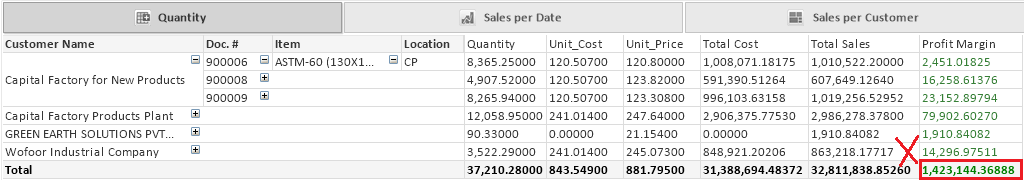New to QlikView

Discussion board where members can get started with QlikView.

Announcements
QlikWorld 2020: Join us May 11 - 14, 2020 in Phoenix, AZ. Register early and save \$400. Learn More
Contributor II

Calculation in pivot table

why the calculation in pivot table is not accurate it give a wrong number, but in straight table it's right !!1 Solution

Accepted Solutions
Valued Contributor II

Re: Calculation in pivot table

hi there,

It depends on what is the level of your aggrigation,

use the following exp

=sum(aggr(

((sum(QTY) + (vQty2)) * (sum([Unit Price])* (1+vSales2/100)))

-

(

((sum([Unit Cost]) * sum(QTY))) * (1+vCost2/100)

)

,

[Customer Name],

[SOP Number],

Item_Description,

[S_Location Code]

))

6 RepliesMVP

Re: Calculation in pivot table

Provide sample application or sample data to work...

Contributor II

qlik example

Contributor II

Re: Calculation in pivot table

I put it in last comment.

regards.MVP

Re: Calculation in pivot table

There are so many information and objects in your qvw. We need to have only those two where you are trying to match!

Valued Contributor II

Re: Calculation in pivot table

hi there,

It depends on what is the level of your aggrigation,

use the following exp

=sum(aggr(

((sum(QTY) + (vQty2)) * (sum([Unit Price])* (1+vSales2/100)))

-

(

((sum([Unit Cost]) * sum(QTY))) * (1+vCost2/100)

)

,

[Customer Name],

[SOP Number],

Item_Description,

[S_Location Code]

))

Contributor II

Thanks a lot.